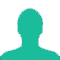# 12 chairs are arranged in a row and are numbered 1 to 12. 4 men have to be seated in these chairs so that the chairs numbered 1 to 8 should be occupied and no two men occupy adjacent chairs. Find the number of ways the task can be done.

A. 360

B. 384

C. 432

D. 470

### Solution(By Examveda Team)

Given there are 12 numbered chairs, such that chairs numbered 1 to 8 should be occupied.
1, 2, 3, 4, 5, 6, 7, 8, 9, 10, 11, 12
The various combinations of chairs that ensure that no two men are sitting together are listed.
(1, 3, 5, __ ), The fourth chair can be 5,6,10,11 or 12, hence 5 ways.
(1, 4, 8, __ ), The fourth chair can be 6,10,11 or 12 hence 4 ways.
(1, 5, 8, __ ), the fourth chair can be 10,11 or 12 hence 3 ways.
(1, 6, 8, __ ), the fourth chair can be 10,11 or 12 hence 3 ways.
(1, 8, 10, 12) is also one of the combinations.
Hence, 16 such combinations exist.
In case of each these combinations we can make the four men inter arrange in 4! ways.
Hence, the required result =16 × 4! = 384

This Question Belongs to Arithmetic Ability >> Permutation And Combination

1.This is a poor quality question from non-GMAT source. I wouldn't worry about it at all but if still interested the solution is as follows.

First of all, I think the question means that "the chairs numbered 1 AND 8 should be occupied".

So, we have that the chairs numbered 1 AND 8 should be occupied and no two adjacent chairs must be occupied. Notice that chairs #2, 7, and 9 cannot be occupied by any of the men (because no two adjacent chairs must be occupied.).

*1*-'2'-3-4-5-6-'7'-*8*-'9'-10-11-12

If the third man occupy chair #3, then the fourth man has 5 options: 5, 6, 10, 11, or 12;
If the third man occupy chair #4, then the fourth man has 4 options: 6, 10, 11, or 12;
If the third man occupy chair #5, then the fourth man has 3 options: 10, 11, or 12;
If the third man occupy chair #6, then the fourth man has 3 options: 10, 11, or 12;
If the third man occupy chair #10, then the fourth man has 1 options: 12.

Total of 5+4+3+3+1=16 cases. For each case the 4 men can be arranged in 4! ways, thus the total number of arrangements is 16*4!=384.

2.